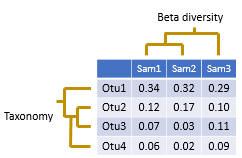Beta diversitySee also

Diversity analysis
Beta diversity metrics
beta_div command

Beta diversity compares two sample. Usually, this is done by calculating a number that indicates the similarity or difference between the samples. Often, but not always, the number is in the range zero to one.

The pair-wise comparisons for a set of samples can be presented in a distance matrix.

In usearch, beta diversities are always differences measures, not similarity measures, so increasing values indicate lower similarity and increasing distance. For distance measures D that ranges between zero and one there is always an equivalant similarity measure S defined by S = 1 – D, for example (Jaccard similarity) = 1 – (Jaccard distance). You can easily convert between distance and similarity measures in a spreadsheet program such as Excel.

Samples can be clustered to bring similar samples together, producing a tree, as shown in the figure above. In usearch, this can be done using the beta_div command, which automatically generates sample trees, or by using the cluster_aggd command to cluster a distance matrix generated by beta_div or by third-party software.